# S5E3 - Gas Law Examples and Practice Problems

In this video blog post, we'll do several gas law practice problems.  The first two examples only contain "1 situation."

These are Ideal Gas Law conditions -- only 1 situation.

## Ideal Gas Law Examples

ex A sample of H2 gas occupies a volume of 8.56 L at a temperature of 0°C and a pressure of 1.5 atm.  How many moles of hydrogen are present?
_________

➞  PV = nRT   ➞  n = PV / RT

➞  n  =  (1.5 atm) (8.56 L)  /  (0.0821 L.atm/K.mol) (273 K)

➞  n  =  0.57 mol H2

Here's the same solution, but with a natural flow...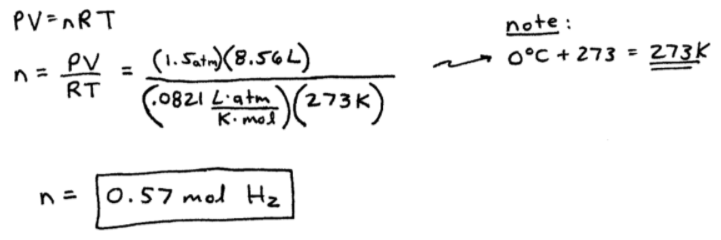----------

ex:  Calculate the volume of 0.845 mol of nitrogen gas at a pressure of 699.2 torr and a temperature of 315 K.
_________

➞  we have to convert pressure from torr to atm

➞  given:

n = 0.845 mol N2
T = 315 K
V = ?
P = 699.2 torr

(699.2 torr / 1) . (1 atm / 760 torr)  =  0.9200 atm

➞  PV = nRT      ➞  V = nRT / P

➞  V = nRT / P   =   (0.845)(0.0821)(315) / 0.9200  =  23.8 L N2

And, here's an easier-to-follow view of the above process: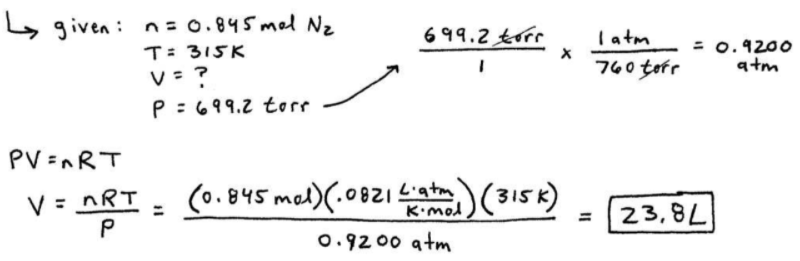----------

TIP:

PV = nRT problems don't typically have a "situation 1" and "situation 2" occurring.  There's usually just one situation.

"Before / After" type problems usually require Boyle's Law, Charles' Law, Avogadro's Law, Gay-Lussac's Law, or the Combined Gas Law.

Let's try some!...

==========

## Charles' Law Example

ex:  A sample of methane gas with a volume of 38 mL at 5°C is heated to 86°C at constant pressure.  Calculate its new volume.
_________

➞  V1 = 38 mL  ,  T1 = 5 + 273 = 278 K

➞  V2 = ?? mL  ,  T2 = 86 + 273 = 359 K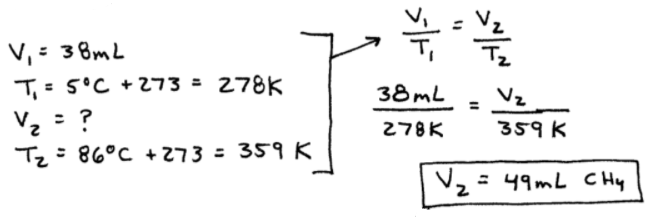... How come it wasn't necessary to convert V1 and V2 into liters (L) ?

Because "R" (in PV = nRT) is equal to 0.0821 L.atm / K.mol, but "R" is not being used in Charles' Law !!  :-)

==========

## Combined Gas Law Example

ex:  Diborane gas (B2H6) has a pressure of 345 torr at a temperature of -15°C and a volume of 3.48 L.  If conditions change such that the temperature is 36°C and the pressure is 468 torr, what will be the volume of the sample?
_________

situation 1:
P1 = 345 torr
T1 = -15 + 273 = 258 K
V1 = 3.48 L

situation 2:
P2 = 468 torr
T2 = 36 + 273 = 309 K
V2 = ?? L

➞  we'll use the Combined Gas Law,

P1V1 / T1  =  P2V2 / T2

V2 = 3.07 L  B2H6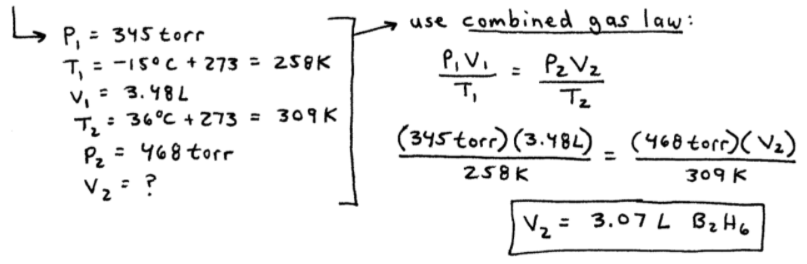==========

## Ideal Gas Law Practice Problem

ex:  A gaseous sample contains 0.35 moles of Argon at a temperature of 13°C and a pressure of 568 torr.  If it's heated to 56°C and a pressure of 897 torr, calculate the change in volume that occurs.
_________

➞  this one's tricky because at first we see two pressures...

However,  n1 = n2 = remains unchanged and we're asked to find the change in volume (ΔV).

To find ΔV = V2 - V1, we'll need to use PV = nRT twice...We will need to convert our pressures from torr to atmospheres (atm), and then set-up two separate PV = nRT equations:

P1V1 = nRT1    and    P2V2 = nRT2

Here's the work that's involved...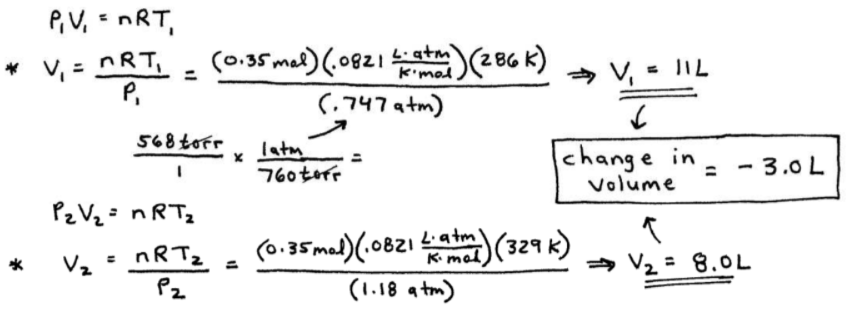==========

That's it for entry #3 from SECTION 5 - Gases.

In my next video from this Section ("Chapter"), we'll discuss Gas Stoichiometry and how to derive Molar Mass and Density from the Ideal Gas Law.